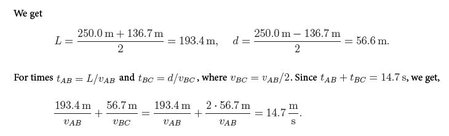Physics

# Motion in 1 Dimension: Mathematical Examples

Example 1: Emmy starts at time t = 0 and drives along a straight path: she starts at point A, drives straight to point B, turns aorund and drives to point C. She ends up at the point at time t = 14.7 s. Her average speed is 17.0 m/s, and the average velocity is 9.3 m/s. a) Find distances between points A and B and points B and C. b) Emmy drives twice as fast from A to B than she drives from B to C. Find her respective speeds.

Solution: We have the distance L between A and B and the distance d between B and C: then

L + d = 17.0 (m/s) x 14.7 s = 250.0m,

L – d = 9.3 x 14.7 s = 136.7 m.We get,

VAB = (193.4 m + 2 x 56.7m) / 17.7 s = 20.9 m/s

And VBC = 10.4 m/s

Example 2: A jaguar can reach speeds of 30 m/s. The fastest person is capable of reaching a speed of 10 m/s. Suppose the the person and the jaguar are 500 m apart. Assume that they are both constantly at their top speed. How long does it take the jaguar to catch up to the person?

Solution: We choose a coordinate system with x = 0 at the person’s starting position so that x = 500 m for the initial position of the jaguar. We find the time-dependent coordinates of the person and jaguar:

Xp(t) = 0 – 10 m/s * t, (person),

Xj(t) = 500 m — 30 m/s * t, (jaguar).

The jaguar catches up to the man when xp = xj so that

-10 m/s * t= 500m— 30 m/s * t,

So, t =  500m / 20 (m/s) = 25.0 s

Discussion: Key to solving kinematics problems is often setting up equations for the coordinates of one or more objects [e.g., the person and the jaguar], and then finding an equation for a particular event [e.g., jaguar catching up to the person].